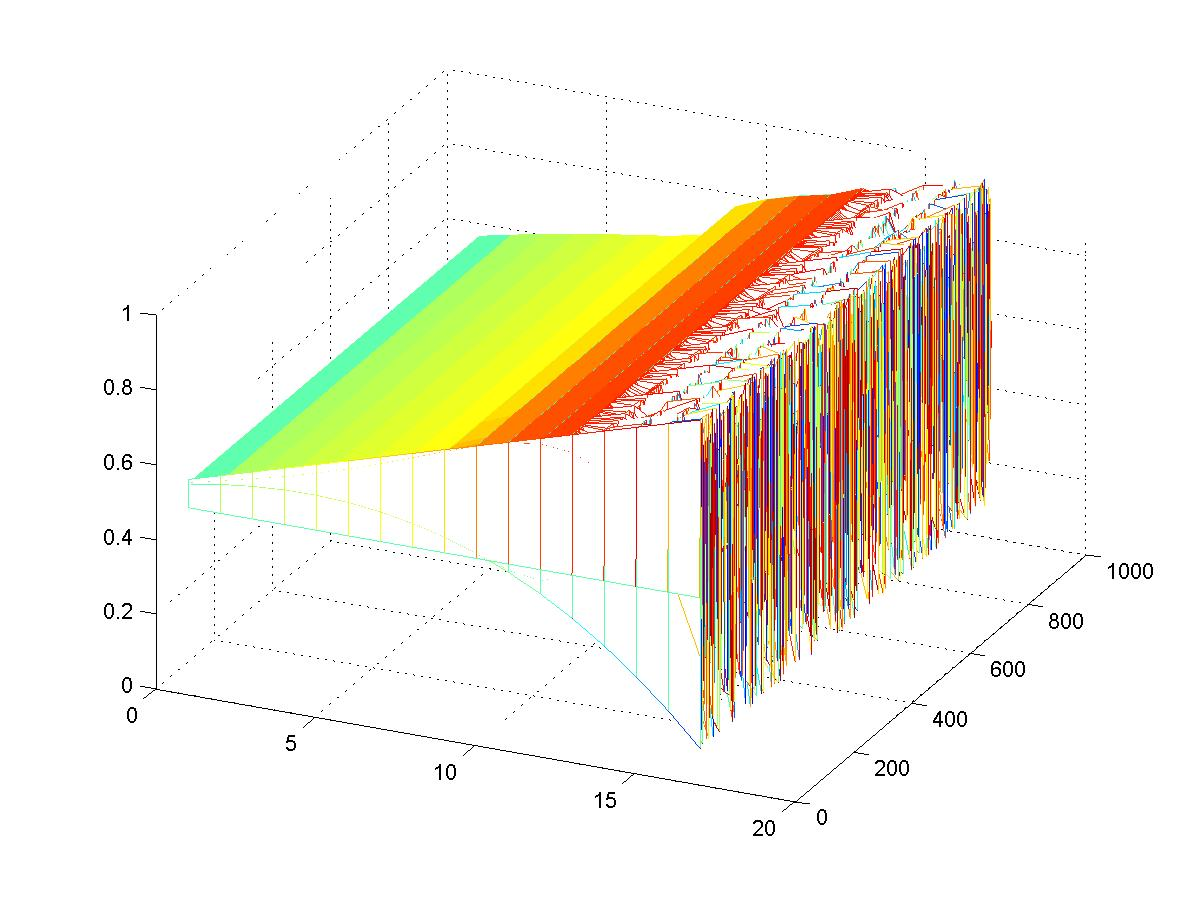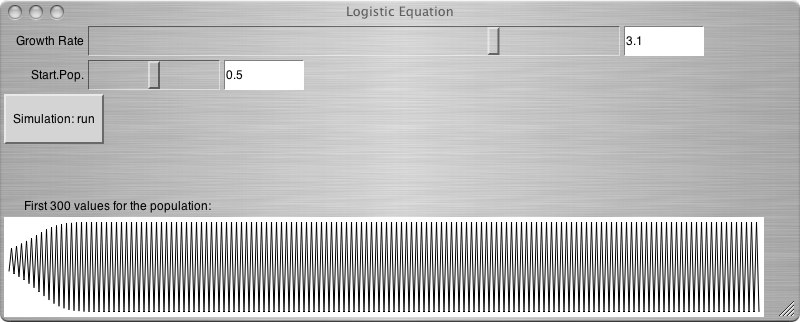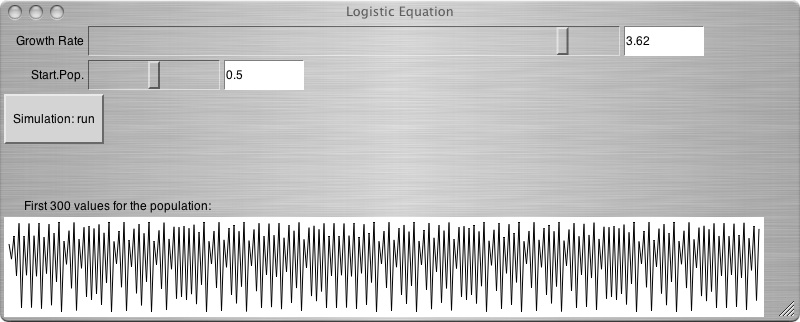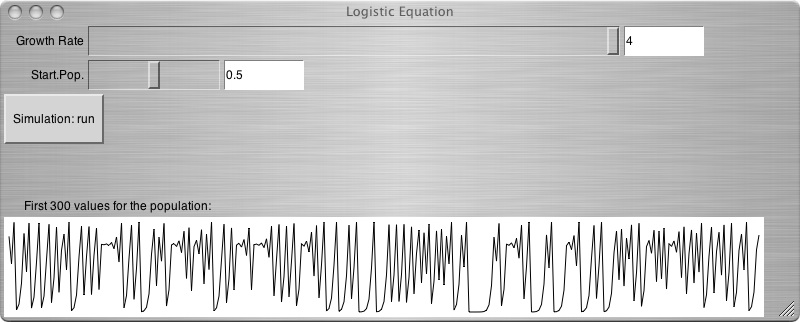# The SonEnvir project portal

##### Personal tools
You are here: Home data Logistic Equation

# Logistic Equation

### Introduction

This short project is an example of how sonification can help observing complex systems.

The logistic equation is a formula for approximating the evolution of a population. The main variable in this equation is the growth rate determining in which type of behaviour the equation settles after a certain period of time. Three types of behaviour are possible:

1. Fixed - the population reaches a stable value
2. Periodic - the population alternated between different values
3. Chaotic - the alternation turns chaoticThe given dataset iterates over 15 different growth rates providing the full range of behaviours of the logistic equation. The task within this data set is to classify the states, which can be difficult with visual representations because hidden periodicities are hard to detect in graphs.

We did a sonification that uses the fluctuations of the population as input and modulates a sine wave with it. The fixed case is trivial, so we concentrated on periodic, quasi-periodic and chaotic settings. These are shown in the following graphs:### Audio examples

• In LogEq-2states.mp3 the population flutuates between two fixed values.
• In LogEq-multistate.mp3 there are numerous states, the system behaves quasi periodic.
• Finally, LogEq-chaos.mp3 gives the noisy sound of a chaotic setting.

### SuperCollider code example

The code example for this sonification (including a simple implementation of the logistic equation) is given here .